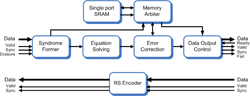Products » Cable Satellite Terrestrial Codecs General » Reed-Solomon codecs »Block diagram

# Reed-Solomon decoder,Reed-Solomon errors only

### Product codes: CMS0013, CMS0007

A Reed-Solomon Codec core for use in many wireless applications including 802.16 and DVB-T/DVB-C/DVB-S.
The Reed-Solomon code has message length K and codeword length N. The symbols for the code are integers between 0 and 2M-1, which represent elements of the finite field GF(2M). The difference N - K must be an even integer. An (N, K) Reed-Solomon code can correct up to T=(N-K)/2 byte (symbol) errors in each codeword.
Commsonic offers two RS Codec products - a full-featured Errors and Erasures Codec(CMS0013) and an Errors-Only Codec(CMS0007).
The Errors and Erasures Codec is intended for applications such as IEEE802.16 that require code puncturing (dynamic control over the number of parity symbols that are used by the code) or with systems such as DVB-MPE that use erased symbols (symbols where the decode confidence is low).
The Errors-Only Codec does not support code puncturing or erased symbols and is optimized to provide a compact design for application that require a fixed number of parity symbols such as MPEG/DVB-T/DVB-S/DVB-C.
A typical application of this core would be for the 802.16 wireless MAN standard where the options would be set as follows:
N=255, K=239, T=8, GF(256) i.e. M=8.
G(x)=(x-α0)(x-α1) … (x-α2T-1).
α=2, J0=0.
P(x) = x8 + x4 + x3 + x2 + 1.
A RS encoder is also available.

## Block diagramClick on diagram to enlarge

#### Synthesis options

• Symbol Size (M): Usually 8-bit.
• Maximum number of correctable errors per packet: Tmax.
• Field Generator or Primitive Polynomial: P(x).
• RS Code generator polynomial: G(x)=(x-αJ0+0)(x-αJ0+1) … (x-αJ0+2T-1).
• Value of primitive root: α.
• Value of integer J0.
• Erasure Enable - synthesizes the logic required to decode erased symbols. †
• Variable T - synthesizes the logic required for codes shortened by puncturing check symbols. †

#### Register settings

• N (up to 2M-1 bits per symbol).
• T (up to Tmax). †

#### Dynamic packet size handling

• A valuable feature in 802.16 and other applications is the ability to manage new N and T settings for each packet that arrives. The N and T parameters are loaded at the start of packet and can be changed immediately afterwards if the following packet requires a new configuration.
† denotes Errors and Erasures codec only (CMS0013).